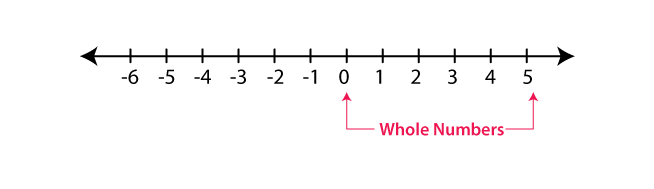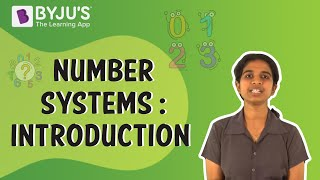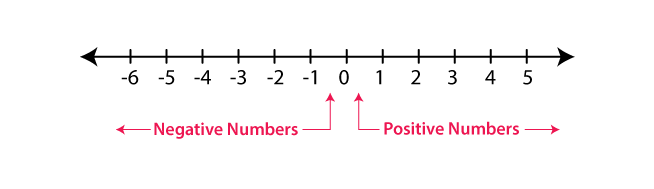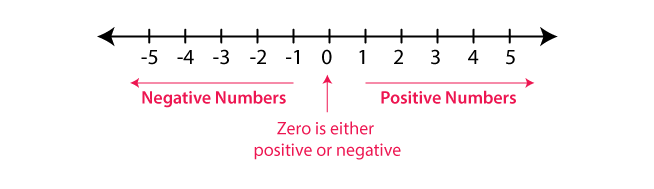# Integers Class 6 Notes Chapter 6

## Introduction

### Whole Numbers

• Whole numbers include zero and all natural numbers, i.e., 0, 1, 2, 3, 4, and so on.To know more about Whole Numbers, visit here.

### Negative Numbers

• The numbers with a negative sign and which lies to the left of zero on the number line are called negative numbers.To know more about Application of Negative Numbers in Daily Life, visit here.

## Introduction to Zero

### The Number Zero

• The number zero means an absence of value.

## The Number Line

### Integers

• Collection of all positive and negative numbers including zero are called integers. ⇒ Numbers …, – 4, – 3, – 2, – 1, 0, 1, 2, 3, 4, … are integers.

### Representing Integers on the Number Line• Draw a line and mark a point as 0 on it
• Points marked to the left (-1, -2, -3, -4, -5, -6) are called negative integers.
• Points marked to the right (1, 2, 3, 4, 5, 6) or (+1, +2, +3, +4, +5, +6) are called positive integers.

### Absolute Value of an Integer

• Absolute value of an integer is the numerical value of the integer without considering its sign.
• Example: Absolute value of -7 is 7 and of +7 is 7.

### Ordering Integers

• On a number line, the number increases as we move towards right and decreases as we move towards left.
• Hence, the order of integers is written as…, –5, –4, – 3, – 2, – 1, 0, 1, 2, 3, 4, 5…
• Therefore, – 3 < – 2, – 2 < – 1, – 1 < 0, 0 < 1, 1 < 2 and 2 < 3.

Positive integer + Negative integer

• Example: (+5) + (-2) Subtract: 5 – 2 = 3 Sign of bigger integer (5): + Answer: +3
• Example: (-5) + (2) Subtract: 5-2 = 3 Sign of the bigger integer (-5): – Answer: -3

Positive integer + Positive integer

• Example: (+5) + (+2) = +7

Negative integer + Negative integer

• Example: (-5) + (-2) = -7

## Properties of Addition and Subtraction of Integers

### Operations on Integers

Operations that can be performed on integers:

• Subtraction
• Multiplication
• Division.

### Subtraction of Integers

• The subtraction of an integer from another integer is same as the addition of the integer and its additive inverse.
• Example: 56 – (–73) = 56 + 73 = 129 and 14 – (8) = 14 – 8 = 6

### Properties of Addition and Subtraction of Integers

• a + b and a – b are integers, where a and b are any integers.

Commutativity Property

• a + b = b + a for all integers a and b.

• (a + b) + c = a + (b + c) for all integers a, b and c.

• Additive Identity is 0, because adding 0 to a number leaves it unchanged.
• a + 0 = 0 + a = a for every integer a.

### Multiplication of Integers

• Product of a negative integer and a positive integer is always a negative integer. 10×−2=−20
• Product of two negative integers is a positive integer. −10×−2=20
• Product of even number of negative integers is positive. (−2)×(−5)=10
• Product of an odd number of negative integers is negative. (−2)×(−5)×(6)=−60

### Properties of Multiplication of Integers

Closure under Multiplication

• Integer * Integer = Integer

Commutativity of Multiplication

• For any two integers a and b, a × b = b × a.

Associativity of Multiplication

• For any three integers a, b and c, (a × b) × c = a × (b × c).

Distributive Property of Integers

• Under addition and multiplication, integers show the distributive property.
• For any integers a, b and c, a × (b + c) = a × b + a × c.

Multiplication by Zero

• For any integer a, a × 0 = 0 × a = 0.

Multiplicative Identity

• 1 is the multiplicative identity for integers.
• a × 1 = 1 × a = a

### Division of Integers

• (positive integer/negative integer)or(negative integer/positive integer)
⇒ The quotient obtained is a negative integer.
• (positive integer/positive integer)or(negative integer/negative integer)
⇒ The quotient obtained is a positive integer.

### Properties of Division of Integers

For any integer a,

• a/0 is not defined
• a/1=a

Integers are not closed under division.

Example: (–9)÷(–3)=3 result is an integer but (−3)÷(−9)=−3−9=13=0.33 which is not an integer.
To know more about Number Lines, visit here.

### Also Check:

Keep visiting BYJU’S for more such CBSE Class 6 notes of maths and science along with other important topic-wise study materials.

## Frequently Asked Questions on CBSE Class 6 Maths Notes Chapter 6 Integers

Q1

### What is ‘Closure property’?

Closure property of whole numbers under addition: The sum of any two whole numbers will always be a whole number, i.e. if a and b are any two whole numbers, a + b will be a whole number.

Q2

### What are the properties of zero?

1. Zero is even 2. Zero is neither positive nor negative 3. Zero is an integer

Q3

### What is the defintion of ‘inverse’?

A term is said to be in inverse proportion to another term if it increases (or decreases) as the other decreases (or increases) of or relating to an inverse function.